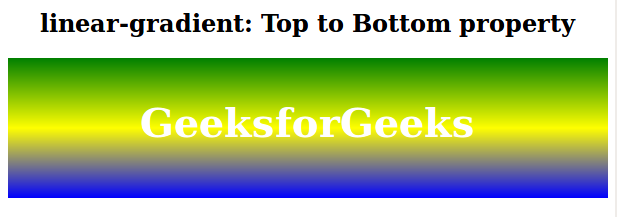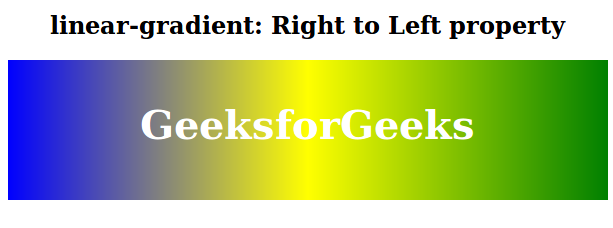The linear-gradient() function is an inbuilt function in CSS that is used to set the linear gradient as the background image.

Syntax:

`background-image: linear-gradient( direction, color1, color2, ... )`

Parameters: This function accepts one direction parameter and many color parameters which are listed below:

• direction: This parameter is used to define the starting point and direction along with the gradient effect.
• color1, color2, …: This parameter is used to hold the color value followed by its optional stop position.

The below examples illustrate the linear-gradient() function in CSS:

Example 1: In this example, The .gradient element applies a linear gradient background from green to yellow to blue, with centered text on top.

## html

 `` `<``html``>`   `<``head``>` `    ``<``title``>linear-gradient function` `    ``<``style``>` `        ``.gradient {` `            ``height: 100px;` `            ``background-image:` `                ``linear-gradient(green, yellow, blue);` `            ``Text-align: center;` `            ``padding-top: 40px;` `            ``font-size: 40px;` `            ``color: white;` `            ``font-weight: bold;` `        ``}`   `        ``h2 {` `            ``text-align: center;` `        ``}` `    ``` ``   `<``body``>` `    ``<``h2``>linear-gradient: Top to Bottom property` `    ``<``div` `class``=``"gradient"``>GeeksforGeeks` ``   ``

Output:Example 2: The .gradient element applies a linear gradient background from right to left, with colors transitioning from green to yellow to blue.

## html

 `` `<``html``>`   `<``head``>` `    ``<``title``>linear-gradient function` `    ``<``style``>` `        ``.gradient {` `            ``height: 100px;` `            ``background-image:` `                ``linear-gradient(to left, green, yellow, blue);` `            ``Text-align: center;` `            ``padding-top: 40px;` `            ``font-size: 40px;` `            ``color: white;` `            ``font-weight: bold;` `        ``}`   `        ``h2 {` `            ``text-align: center;` `        ``}` `    ``` ``   `<``body``>` `    ``<``h2``>` `        ``linear-gradient: Right to Left property` `    ``` `    ``<``div` `class``=``"gradient"``>` `        ``GeeksforGeeks` `    ``` ``   ``

Output:Supported Browser: